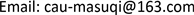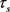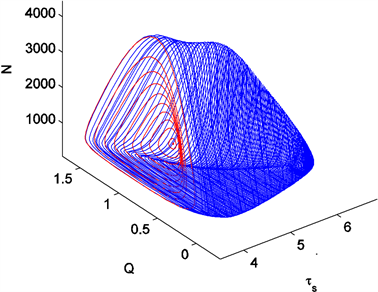﻿ 造血系统的下临界Hopf分岔 Subcritical Hopf Bifurcation of a Hematopological System

Vol.06 No.06(2017), Article ID:22113,12 pages
10.12677/AAM.2017.66089

Subcritical Hopf Bifurcation of a Hematopological System

Suqi Ma

Mathematical Department of Chinese Agricultural University, BeijingReceived: Sep. 1st, 2017; accepted: Sep. 15th, 2017; published: Sep. 21st, 2017ABSTRACT

The periodic cyclical neutrophil oscillating model of hematopoietic is discussed. Mathematically, the model is a multi-delay DDEs with high nonlinearity. Varying time delay brings forth Hopf bifurcation in system dynamic behavior. By calculating coefficients of normal form, it is analyzed that Hopf bifurcation is sub-critical. Numerical simulation coincides with the theoretical analyze result.

Keywords:Hematological Cell Model, Subcritical Hopf Bifurcation, Delay1. 引言

$\begin{array}{l}\frac{\text{d}Q}{\text{d}t}=-\left(\beta \left(Q\right)+{k}_{N}\left(N\right)+{k}_{\delta }\right)Q+2{\text{e}}^{-{\gamma }_{s}{\tau }_{s}}\beta \left({Q}_{{\tau }_{s}}\right){Q}_{{\tau }_{s}}\\ \frac{\text{d}N}{\text{d}t}=-{\gamma }_{N}N+{\text{e}}^{{\eta }_{NP}{\tau }_{NP}-{\gamma }_{0}{\tau }_{NM}}{k}_{N}\left({N}_{{\tau }_{N}}\right){Q}_{{\tau }_{N}}\end{array}$ (1)

$\begin{array}{l}{k}_{N}\left(N\right)=\frac{{f}_{0}{\theta }_{1}^{m}}{{\theta }_{1}^{m}+{N}^{m}},\text{\hspace{0.17em}}\text{\hspace{0.17em}}\beta \left(Q\right)=\frac{{k}_{0}{\theta }_{2}^{n}}{{\theta }_{2}^{n}+{Q}^{n}}\\ {k}_{N}\left({N}_{{\tau }_{N}}\right)=\frac{{f}_{0}{\theta }_{1}^{m}}{{\theta }_{1}^{m}+N{\left(t-{\tau }_{N}\right)}^{m}},\text{\hspace{0.17em}}\text{\hspace{0.17em}}\beta \left(Q\right)=\frac{{k}_{0}{\theta }_{2}^{n}}{{\theta }_{2}^{n}+Q{\left(t-{\tau }_{s}\right)}^{n}}\end{array}$

(1) 造血过程由激发分化和成熟机制组成，具体地，造血干细胞经由细胞分裂分化成为全部血液细胞包括白细胞、红细胞和血小板，并能自我更新， ${\gamma }_{s}$ 表示凋亡率，增殖相需要的天数是(2) 与嗜中性粒细胞不同的是，血液干细胞分化以速率 ${k}_{\delta }$ 分化成为其它血液细胞；

(3) 嗜中性粒细胞耦合时滞为 ${\tau }_{N}$ ，由两部分和组成，分别是其前驱细胞的增殖相需要的天数 ${\tau }_{NP}$ 和成熟相需要的天数 ${\tau }_{NM}$${\eta }_{NP}$ 是增殖速率， ${\gamma }_{0}$ 是成熟相中的死亡率。因而，嗜中性粒细胞的组合相中的放大系数为

${A}_{N}={\text{e}}^{{\eta }_{NP}{\tau }_{NP}-{\gamma }_{0}{\tau }_{NM}}$

2. Hopf分岔分析

$\left({Q}_{0},{N}_{0}\right)$ 是系统(1)的平衡点，计算方程在平衡点的特征值，如果方程(1)的特征方程有一对纯虚根 $±i{\omega }_{0}$ ，并满足横截条件，则方程(1)发生了Hopf分岔，且经由Hopf分岔，系统(1)出现稳定或不稳定的周期解。作坐标平移，系统的三次Taylor截断展开式为

$\begin{array}{l}{x}^{\prime }={a}_{11}x+{a}_{12}y+{b}_{1}x\left(t-{\tau }_{s}\right)+f\left(x,x\left(t-{\tau }_{s}\right),y\right)\\ {y}^{\prime }=-{\gamma }_{N}y+{b}_{2}x\left(t-{\tau }_{N}\right)+{b}_{3}y\left(t-{\tau }_{N}\right)+g\left(x\left(t-{\tau }_{N}\right),y,y\left(t-{\tau }_{N}\right)\right)\end{array}$

$\begin{array}{l}{a}_{11}=-\beta \left({Q}^{*}\right)-{k}_{N}\left({N}^{*}\right)-{k}_{\delta }-{\beta }^{\prime }\left({Q}^{*}\right){Q}^{*},\text{\hspace{0.17em}}\text{\hspace{0.17em}}\text{\hspace{0.17em}}{a}_{12}=-{{k}^{\prime }}_{N}\left({N}^{*}\right){Q}^{*},\\ {b}_{1}=2{\text{e}}^{-{r}_{s}{\tau }_{s}}\left(\beta \left({Q}^{*}\right)+{\beta }^{\prime }\left({Q}^{*}\right){Q}^{*}\right),\text{\hspace{0.17em}}\text{\hspace{0.17em}}\text{\hspace{0.17em}}{b}_{2}={\text{e}}^{{\tau }_{NP}{\eta }_{NP}-{\gamma }_{0}{\tau }_{NM}}k\left({N}^{*}\right),\\ {b}_{3}={\text{e}}^{{\tau }_{NP}{\eta }_{NP}-{\gamma }_{0}{\tau }_{NM}}{k}^{\prime }\left({N}^{*}\right){Q}^{*},\end{array}$

$\begin{array}{c}f\left(x,x\left(t-{\tau }_{s}\right),y\right)=-{\beta }^{\prime }\left({Q}^{*}\right){x}^{2}-\frac{1}{2}{\beta }^{″}\left({Q}^{*}\right){Q}^{*}{x}^{2}-{{k}^{\prime }}_{N}\left({N}^{*}\right)xy-\frac{1}{2}{{k}^{″}}_{N}\left({N}^{*}\right){Q}^{*}{y}^{2}\\ \text{\hspace{0.17em}}\text{\hspace{0.17em}}+2{\text{e}}^{-{\gamma }_{s}{\tau }_{s}}{\beta }^{\prime }\left({Q}^{*}\right){x}^{2}\left(t-{\tau }_{s}\right)+{\text{e}}^{-{\gamma }_{s}{\tau }_{s}}{\beta }^{″}\left({Q}^{*}\right){Q}^{*}{x}^{2}\left(t-{\tau }_{s}\right)\\ \text{\hspace{0.17em}}\text{\hspace{0.17em}}+{\text{e}}^{-{\gamma }_{s}{\tau }_{s}}{\beta }^{″}\left({Q}^{*}\right){x}^{3}\left(t-{\tau }_{s}\right)-\frac{1}{2}{\beta }^{″}\left({Q}^{*}\right){x}^{3}-\frac{1}{6}{\beta }^{‴}\left({Q}^{*}\right){Q}^{*}{x}^{3}-\frac{1}{2}{{k}^{″}}_{N}\left({N}^{*}\right)x{y}^{2}\\ \text{\hspace{0.17em}}\text{\hspace{0.17em}}-\frac{1}{6}{{k}^{‴}}_{N}\left({N}^{*}\right){Q}^{*}{y}^{3}+{\text{e}}^{-{r}_{s}{\tau }_{s}}{\beta }^{″}\left({Q}^{*}\right){x}^{3}\left(t-{\tau }_{s}\right)+\frac{1}{3}{\text{e}}^{-{r}_{s}{\tau }_{s}}{\beta }^{‴}\left({Q}^{*}\right){Q}^{*}{x}^{3}\left(t-{\tau }_{s}\right)\\ \text{\hspace{0.17em}}\text{\hspace{0.17em}}+o\left(‖{x}^{3}‖,‖{x}^{3}\left(t-{\tau }_{s}\right)‖,‖{y}^{3}‖\right)\end{array}$

$\begin{array}{l}g\left(x\left(t-{\tau }_{N}\right),y,y\left(t-{\tau }_{N}\right)\right)\\ ={\text{e}}^{{\tau }_{NP}{\eta }_{NP}-{\gamma }_{0}{\tau }_{NM}}{{k}^{\prime }}_{N}\left({N}^{*}\right)x\left(t-{\tau }_{N}\right)y\left(t-{\tau }_{N}\right)\\ \text{\hspace{0.17em}}\text{\hspace{0.17em}}\text{ }\text{ }+\frac{1}{2}{\text{e}}^{{\tau }_{NP}{\eta }_{NP}-{\gamma }_{0}{\tau }_{NM}}{{k}^{″}}_{N}\left({N}^{*}\right){Q}^{*}{y}^{2}\left(t-{\tau }_{N}\right)+\frac{1}{2}{\text{e}}^{{\tau }_{NP}{\eta }_{NP}-{\gamma }_{0}{\tau }_{NM}}{{k}^{″}}_{N}\left({N}^{*}\right)x\left(t-{\tau }_{N}\right){y}^{2}\left(t-{\tau }_{N}\right)\\ \text{\hspace{0.17em}}\text{\hspace{0.17em}}\text{ }\text{ }+\frac{1}{6}{\text{e}}^{{\tau }_{NP}{\eta }_{NP}-{\gamma }_{0}{\tau }_{NM}}{{k}^{‴}}_{N}\left({N}^{*}\right){Q}^{*}{y}^{3}\left(t-{\tau }_{N}\right)+\frac{1}{6}{\text{e}}^{{\tau }_{NP}{\eta }_{NP}-{\gamma }_{0}{\tau }_{NM}}{{k}^{‴}}_{N}\left({N}^{*}\right)x\left(t-{\tau }_{N}\right){y}^{3}\left(t-{\tau }_{N}\right)\end{array}$

$\begin{array}{c}{m}^{\prime }\left(t\right)=L\left(\mu \right)m\left(t\right)+{R}_{1}\left(\mu \right)m\left(t-{\tau }_{s}\right)+{R}_{2}\left(\mu \right)m\left(t-{\tau }_{N}\right)\\ \text{\hspace{0.17em}}\text{\hspace{0.17em}}+F\left(m\left(t\right),m\left(t-{\tau }_{s}\right),m\left(t-{\tau }_{N}\right),\mu \right)\end{array}$ (2)

$L=\left(\begin{array}{cc}a{}_{11}& {a}_{12}\\ 0& -{\gamma }_{N}\end{array}\right)$ , ${R}_{1}=\left(\begin{array}{cc}{b}_{1}& 0\\ 0& 0\end{array}\right)$ , ${R}_{2}=\left(\begin{array}{cc}0& 0\\ {b}_{2}& {b}_{3}\end{array}\right)$

$\begin{array}{l}F\left(m\left(t\right),m\left(t-{\tau }_{s}\right),m\left(t-{\tau }_{N}\right),\mu \right)\\ =\frac{1}{2}B\left(m\left(t\right),m\left(t\right)\right)+\frac{1}{2}\stackrel{˜}{B}\left(m\left(t-{\tau }_{s}\right),m\left(t-{\tau }_{s}\right)\right)\\ \text{\hspace{0.17em}}\text{\hspace{0.17em}}+\frac{1}{2}\stackrel{^}{B}\left(m\left(t-{\tau }_{N}\right),m\left(t-{\tau }_{N}\right)\right)+\frac{1}{6}C\left(m\left(t\right),m\left(t\right),m\left(t\right)\right)\\ \text{\hspace{0.17em}}\text{\hspace{0.17em}}+\frac{1}{6}\stackrel{˜}{C}\left(m\left(t-{\tau }_{s}\right),m\left(t-{\tau }_{s}\right),m\left(t-{\tau }_{s}\right)\right)+\frac{1}{6}\stackrel{^}{C}\left(m\left(t-{\tau }_{N}\right),m\left(t-{\tau }_{N}\right),m\left(t-{\tau }_{N}\right)\right)\end{array}$ (3)

$C$ 表示连续可微函数 $u:\left[-{\tau }_{N},0\right]\to {R}^{2}$ 构成的巴拿赫空间，定义时间平移 ${u}_{t}\left(\theta \right)=m\left(t+\theta \right)$ , $\theta \in \left[-{\tau }_{N},0\right]$ ，那么方程(2)的算子微分方程形式为

${{m}^{\prime }}_{t}=A{m}_{t}+F\left({m}_{t}\right)$ (4)

$Au\left(\theta \right)=\left\{\begin{array}{l}\frac{\text{d}}{\text{d}\theta }u\left(\theta \right),\text{\hspace{0.17em}}\text{\hspace{0.17em}}\text{\hspace{0.17em}}\text{\hspace{0.17em}}\text{\hspace{0.17em}}\text{\hspace{0.17em}}\text{\hspace{0.17em}}\text{\hspace{0.17em}}\text{\hspace{0.17em}}\text{\hspace{0.17em}}\text{\hspace{0.17em}}\text{\hspace{0.17em}}\text{\hspace{0.17em}}\text{\hspace{0.17em}}\text{\hspace{0.17em}}\text{\hspace{0.17em}}\text{\hspace{0.17em}}\text{\hspace{0.17em}}\text{\hspace{0.17em}}\text{\hspace{0.17em}}\text{\hspace{0.17em}}\text{\hspace{0.17em}}\text{\hspace{0.17em}}\text{\hspace{0.17em}}\text{\hspace{0.17em}}\text{\hspace{0.17em}}\text{\hspace{0.17em}}\text{\hspace{0.17em}}\text{\hspace{0.17em}}\text{\hspace{0.17em}}\text{\hspace{0.17em}}\text{ }\text{\hspace{0.17em}}\theta \in \left[-{\tau }_{N},0\right),\\ Lu\left(0\right)+{R}_{1}u\left(-{\tau }_{s}\right)+{R}_{2}u\left(-{\tau }_{N}\right),\text{\hspace{0.17em}}\text{\hspace{0.17em}}\theta =0,\end{array}$ (5)

$H\left(u\right)\left(\theta \right)=\left\{\begin{array}{l}0,\text{\hspace{0.17em}}\text{\hspace{0.17em}}\text{\hspace{0.17em}}\text{\hspace{0.17em}}\text{\hspace{0.17em}}\text{\hspace{0.17em}}\text{\hspace{0.17em}}\text{\hspace{0.17em}}\text{\hspace{0.17em}}\text{\hspace{0.17em}}\text{\hspace{0.17em}}\text{\hspace{0.17em}}\text{\hspace{0.17em}}\text{\hspace{0.17em}}\text{\hspace{0.17em}}\text{\hspace{0.17em}}\text{\hspace{0.17em}}\text{\hspace{0.17em}}\text{\hspace{0.17em}}\text{\hspace{0.17em}}\text{\hspace{0.17em}}\text{\hspace{0.17em}}\text{\hspace{0.17em}}\text{\hspace{0.17em}}\text{\hspace{0.17em}}\text{\hspace{0.17em}}\text{\hspace{0.17em}}\text{\hspace{0.17em}}\text{\hspace{0.17em}}\text{\hspace{0.17em}}\text{\hspace{0.17em}}\text{\hspace{0.17em}}\text{\hspace{0.17em}}\text{\hspace{0.17em}}\text{\hspace{0.17em}}\text{\hspace{0.17em}}\text{\hspace{0.17em}}\text{\hspace{0.17em}}\theta \in \left[-{\tau }_{N},0\right),\\ F\left(u\left(0\right),u\left(-{\tau }_{s}\right),u\left(-{\tau }_{N}\right)\right),\text{\hspace{0.17em}}\text{\hspace{0.17em}}\text{\hspace{0.17em}}\theta =0,\end{array}$ (6)

${A}^{*}v\left(s\right)=\left\{\begin{array}{l}-\frac{\text{d}}{\text{d}s}v\left(s\right),\text{\hspace{0.17em}}\text{\hspace{0.17em}}\text{\hspace{0.17em}}\text{\hspace{0.17em}}\text{\hspace{0.17em}}\text{\hspace{0.17em}}\text{\hspace{0.17em}}\text{\hspace{0.17em}}\text{\hspace{0.17em}}\text{\hspace{0.17em}}\text{\hspace{0.17em}}\text{\hspace{0.17em}}\text{\hspace{0.17em}}\text{\hspace{0.17em}}\text{\hspace{0.17em}}\text{\hspace{0.17em}}\text{\hspace{0.17em}}\text{\hspace{0.17em}}\text{\hspace{0.17em}}\text{\hspace{0.17em}}\text{\hspace{0.17em}}\text{\hspace{0.17em}}\text{\hspace{0.17em}}\text{\hspace{0.17em}}\text{\hspace{0.17em}}\text{\hspace{0.17em}}\text{\hspace{0.17em}}\text{\hspace{0.17em}}\text{\hspace{0.17em}}\text{\hspace{0.17em}}\text{\hspace{0.17em}}s\in \left(0,{\tau }_{N}\right)\\ {L}^{\text{T}}v\left(0\right)+{R}_{1}^{\text{T}}v\left({\tau }_{s}\right)+{R}_{2}^{\text{T}}v\left({\tau }_{N}\right),\text{\hspace{0.17em}}\text{\hspace{0.17em}}\text{\hspace{0.17em}}\text{\hspace{0.17em}}s=0\end{array}$

$〈v,u〉={\overline{v}}^{\text{T}}\left(0\right)u\left(0\right)+{\int }_{-{\tau }_{s}}^{0}{\overline{v}}^{\text{T}}\left(\xi +{\tau }_{s}\right){R}_{1}u\left(\xi \right)\text{d}\xi +{\int }_{-{\tau }_{N}}^{0}{\overline{v}}^{\text{T}}\left(\xi +{\tau }_{N}\right){R}_{2}u\left(\xi \right)\text{d}\xi$ (7)

$〈v,Au〉=〈{A}^{*}v,u〉$

$C={P}_{\Lambda }\oplus {Q}_{\Lambda }$ ，其中 ${P}_{\Lambda }$ 为相应于 $\Lambda$ 的特征空间，而 ${Q}_{\Lambda }$ 是其补空间。计算得 $A\left(0\right)$ 相应于特征值 $i{\omega }_{0}$ 的特征向量为 $q\left(\theta \right)=\left(\begin{array}{c}{\gamma }_{N}+i{\omega }_{0}-{b}_{3}{\text{e}}^{-i{\omega }_{0}{\tau }_{N}}\\ {b}_{2}{\text{e}}^{-i{\omega }_{0}{\tau }_{N}}\end{array}\right){\text{e}}^{i{\omega }_{0}\theta }$$-{\tau }_{N}\le \theta \le 0$${A}^{*}\left(0\right)$ 相应于 $-i{\omega }_{0}$ 的特征向量为 ${q}^{*}\left(s\right)=l\left(\begin{array}{c}-{b}_{2}{\text{e}}^{i{\omega }_{0}{\tau }_{N}}\\ {a}_{11}+i{\omega }_{0}+{b}_{1}{\text{e}}^{i{\omega }_{0}{\tau }_{s}}\end{array}\right){\text{e}}^{i{\omega }_{0}s}$$0\le s\le {\tau }_{N}$ ,满足 $〈{q}^{*},q〉=1$ ，进一步可证明 $〈{q}^{*},\overline{q}〉=0$

$\begin{array}{l}Aq\left(\theta \right)=i{\omega }_{0}q\left(\theta \right),\text{\hspace{0.17em}}\text{\hspace{0.17em}}\text{\hspace{0.17em}}-{\tau }_{N}\le \theta \le 0,\\ {A}^{*}{q}^{*}\left(s\right)=-i{\omega }_{0}{q}^{*}\left(s\right),\text{\hspace{0.17em}}\text{\hspace{0.17em}}\text{\hspace{0.17em}}0\le s\le {\tau }_{N}\end{array}$ (8)

${u}_{t}=zq+\overline{z}\overline{q}+w$ (9)

$z=〈{q}^{*},{m}_{t}〉,\text{\hspace{0.17em}}\text{\hspace{0.17em}}w\in {Q}_{\Lambda }$ (10)

$\begin{array}{c}{z}^{\prime }\left(t\right)=〈{q}^{*},{{m}^{\prime }}_{t}〉=〈{q}^{*},Azq〉+〈{q}^{*},H\left(zq+\overline{z}\overline{q}+w\left(t\right)\right)〉\\ =i{\omega }_{0}z+{\overline{q}}^{*}\left(0\right){F}^{*}\left(z,\overline{z}\right)\end{array}$ (11)

${F}^{*}\left(z,\overline{z}\right)=F\left(2\mathrm{Re}\left\{zq\left(\theta \right)\right\}+w\left(t\right)\right)$ (12)

3. 二维中心流形

$w=v\left(z,\overline{z}\right)$ 展开成级数

$w=v\left(z,\overline{z}\right)=\left(\begin{array}{c}\sum _{2\le i+j}\frac{{v}_{ij}^{\left(1\right)}}{i!j!}\left(z,\overline{z}\right)\\ \sum _{2\le i+j}\frac{{v}_{ij}^{\left(2\right)}}{i!j!}\left(z,\overline{z}\right)\end{array}\right)$ (13)

${z}^{\prime }\left(t\right)=i{\omega }_{0}z+G\left(z,\overline{z}\right)$ (13)

$G\left(z,\overline{z}\right)=\sum _{2\le i+j}\frac{{G}_{ij}}{i!j!}\left(z,\overline{z}\right)$ (14)

$\frac{\text{d}v}{\text{d}t}=\left\{\begin{array}{l}Av-2\mathrm{Re}\left\{{\overline{q}}^{*}\left(0\right)\stackrel{^}{F}q\left(\theta \right)\right\},\text{\hspace{0.17em}}\text{\hspace{0.17em}}\text{\hspace{0.17em}}\text{\hspace{0.17em}}\text{\hspace{0.17em}}\text{\hspace{0.17em}}\text{\hspace{0.17em}}\text{\hspace{0.17em}}\text{\hspace{0.17em}}\text{\hspace{0.17em}}\text{\hspace{0.17em}}\text{\hspace{0.17em}}-{\tau }_{N}\le \theta <0,\\ Av-2\mathrm{Re}\left\{{\overline{q}}^{*}\left(0\right)\stackrel{^}{F}q\left(\theta \right)\right\}+\stackrel{^}{F},\text{\hspace{0.17em}}\text{\hspace{0.17em}}\text{\hspace{0.17em}}\text{\hspace{0.17em}}\text{\hspace{0.17em}}\text{\hspace{0.17em}}\text{\hspace{0.17em}}\text{\hspace{0.17em}}\text{\hspace{0.17em}}\text{\hspace{0.17em}}\text{\hspace{0.17em}}\theta =0,\end{array}$ (15)

$\frac{\text{d}v}{\text{d}t}=Av\left(z,\overline{z}\right)+R\left(z,\overline{z}\right)$ (16)

$R\left(z,\overline{z}\right)=-2\mathrm{Re}\left\{{\overline{q}}^{*}\left(0\right)\stackrel{^}{F}q\left(\theta \right)\right\}=\left(\begin{array}{c}\frac{{R}_{20}^{\left(1\right)}}{2}{z}^{2}+{R}_{11}^{\left(1\right)}z\overline{z}+\frac{{R}_{02}^{\left(1\right)}}{2}{\overline{z}}^{2}+\cdots \\ \frac{{R}_{20}^{\left(2\right)}}{2}{z}^{2}+{R}_{11}^{\left(2\right)}z\overline{z}+\frac{{R}_{02}^{\left(2\right)}}{2}{\overline{z}}^{2}+\cdots \end{array}\right)$

$\begin{array}{l}-2\mathrm{Re}\left\{{\overline{q}}^{*}\left(0\right)\stackrel{^}{F}q\left(\theta \right)\right\}\\ =-G\left(z,\overline{z}\right)q\left(\theta \right)-\overline{G}\left(z,\overline{z}\right)\overline{q}\left(\theta \right)\\ =-\left(\frac{{G}_{20}}{2}{z}^{2}+{G}_{11}z\overline{z}+\frac{{G}_{02}}{2}{\overline{z}}^{2}\right)q\left(\theta \right)-\left(\frac{{\overline{G}}_{20}}{2}{\overline{z}}^{2}+{\overline{G}}_{11}z\overline{z}+\frac{{\overline{G}}_{02}}{2}{z}^{2}\right)\overline{q}\left(\theta \right)\end{array}$

${R}_{20}^{\left(1\right)}=-{G}_{20}{q}_{1}{\text{e}}^{i{\omega }_{0}\theta }-{\overline{G}}_{02}{\overline{q}}_{1}{\text{e}}^{-i{\omega }_{0}\theta }$ , ${R}_{20}^{\left(2\right)}=-{G}_{20}{q}_{2}{\text{e}}^{i{\omega }_{0}\theta }-{\overline{G}}_{02}{\overline{q}}_{2}{\text{e}}^{-i{\omega }_{0}\theta }$

${R}_{11}^{\left(1\right)}=-{G}_{11}{q}_{1}{\text{e}}^{i{\omega }_{0}\theta }-{\overline{G}}_{11}{\overline{q}}_{1}{\text{e}}^{-i{\omega }_{0}\theta }$ , ${R}_{11}^{\left(2\right)}=-{G}_{11}{q}_{2}{\text{e}}^{i{\omega }_{0}\theta }-{\overline{G}}_{11}{\overline{q}}_{2}{\text{e}}^{-i{\omega }_{0}\theta }$

${R}_{02}^{\left(1\right)}=-{G}_{02}{q}_{1}{\text{e}}^{i{\omega }_{0}\theta }-{\overline{G}}_{20}{\overline{q}}_{1}{\text{e}}^{-i{\omega }_{0}\theta }$ , ${R}_{02}^{\left(2\right)}=-{G}_{02}{q}_{2}{\text{e}}^{i{\omega }_{0}\theta }-{\overline{G}}_{20}{\overline{q}}_{2}{\text{e}}^{-i{\omega }_{0}\theta }$

$\begin{array}{l}A{v}_{20}^{\left(1,2\right)}=2i{\omega }_{0}{v}_{20}^{\left(1,2\right)}-{R}_{20}^{\left(1,2\right)},\\ A{v}_{11}^{\left(1,2\right)}=-{R}_{11}^{\left(1,2\right)},\\ A{v}_{02}^{\left(1,2\right)}=-2i{\omega }_{0}{v}_{02}^{\left(1,2\right)}-{R}_{02}^{\left(1,2\right)}\end{array}$ (17)

$\begin{array}{l}\left\{\begin{array}{l}\frac{\text{d}{v}_{20}^{\left(1\right)}}{\text{d}t}=2i{\omega }_{0}{v}_{20}^{\left(1\right)}+{G}_{20}{q}_{1}{\text{e}}^{i{\omega }_{0}\theta }+{\overline{G}}_{02}{\overline{q}}_{1}{\text{e}}^{-i{\omega }_{0}\theta }\\ \frac{\text{d}{v}_{20}^{\left(2\right)}}{\text{d}t}=2i{\omega }_{0}{v}_{20}^{\left(2\right)}+{G}_{20}{q}_{2}{\text{e}}^{i{\omega }_{0}\theta }+{\overline{G}}_{02}{\overline{q}}_{2}{\text{e}}^{-i{\omega }_{0}\theta }\end{array}\\ \left\{\begin{array}{l}\frac{\text{d}{v}_{11}^{\left(1\right)}}{\text{d}t}={G}_{11}{q}_{1}{\text{e}}^{i{\omega }_{0}\theta }+{\overline{G}}_{11}{\overline{q}}_{1}{\text{e}}^{-i{\omega }_{0}\theta }\\ \frac{\text{d}{v}_{11}^{\left(2\right)}}{\text{d}t}={G}_{11}{q}_{2}{\text{e}}^{i{\omega }_{0}\theta }+{\overline{G}}_{11}{\overline{q}}_{2}{\text{e}}^{-i{\omega }_{0}\theta }\end{array}\\ \left\{\begin{array}{l}\frac{\text{d}{v}_{02}^{\left(1\right)}}{\text{d}t}=-2i{\omega }_{0}{v}_{02}^{\left(1\right)}+{G}_{02}{q}_{1}{\text{e}}^{i{\omega }_{0}\theta }+{\overline{G}}_{20}{\overline{\overline{)q}}}_{1}{\text{e}}^{-i{\omega }_{0}\theta }\\ \frac{\text{d}{v}_{02}^{\left(2\right)}}{\text{d}t}=-2i{\omega }_{0}{v}_{02}^{\left(1\right)}+{G}_{02}{q}_{2}{\text{e}}^{i{\omega }_{0}\theta }+{\overline{G}}_{20}{\overline{q}}_{2}{\text{e}}^{-i{\omega }_{0}\theta }\end{array}\end{array}$$-{\tau }_{N}\le \theta <0$ (18)

$\left\{\begin{array}{l}{a}_{11}{{v}_{20}^{\left(1\right)}|}_{\theta =0}+{a}_{12}{{v}_{20}^{\left(2\right)}|}_{\theta =0}-2i{\omega }_{0}{{v}_{20}^{\left(1\right)}|}_{\theta =0}+{b}_{1}{{v}_{20}^{\left(1\right)}|}_{\theta =-{\tau }_{s}}+{R}_{20}^{\left(1\right)}+2{f}_{0}=0\\ -{\gamma }_{N}{{v}_{20}^{\left(2\right)}|}_{\theta =0}-2i{\omega }_{0}{{v}_{20}^{\left(2\right)}|}_{\theta =0}+{b}_{2}{{v}_{20}^{\left(1\right)}|}_{\theta =-{\tau }_{N}}+{b}_{3}{{v}_{20}^{\left(2\right)}|}_{\theta =-{\tau }_{N}}+{R}_{20}^{\left(2\right)}+2{g}_{0}=0\end{array}$

$\left\{\begin{array}{l}{a}_{11}{{v}_{11}^{\left(1\right)}|}_{\theta =0}+{a}_{12}{{v}_{11}^{\left(2\right)}|}_{\theta =0}+{b}_{1}{{v}_{11}^{\left(1\right)}|}_{\theta =-{\tau }_{s}}+{R}_{11}^{\left(1\right)}+{f}_{1}=0\\ -{\gamma }_{N}{{v}_{11}^{\left(2\right)}|}_{\theta =0}+{b}_{2}{{v}_{11}^{\left(1\right)}|}_{\theta =-{\tau }_{N}}+{b}_{3}{{v}_{11}^{\left(2\right)}|}_{\theta =-{\tau }_{N}}+{R}_{11}^{\left(2\right)}+{g}_{1}=0\end{array}$ (19)

$\left\{\begin{array}{l}{a}_{11}{{v}_{02}^{\left(1\right)}|}_{\theta =0}+{a}_{12}{{v}_{02}^{\left(2\right)}|}_{\theta =0}+2i{\omega }_{0}{{v}_{02}^{\left(1\right)}|}_{\theta =0}+{b}_{1}{{v}_{02}^{\left(1\right)}|}_{\theta =-{\tau }_{s}}+{R}_{02}^{\left(1\right)}+2{f}_{2}=0\\ -{\gamma }_{N}{{v}_{02}^{\left(2\right)}|}_{\theta =0}+2i{\omega }_{0}{{v}_{02}^{\left(2\right)}|}_{\theta =0}+{b}_{2}{{v}_{02}^{\left(1\right)}|}_{\theta =-{\tau }_{N}}+{b}_{3}{{v}_{02}^{\left(2\right)}|}_{\theta =-{\tau }_{N}}+{R}_{02}^{\left(2\right)}+2{g}_{2}=0\end{array}$

$\left\{\begin{array}{l}{v}_{20}^{\left(1\right)}={E}_{1}{\text{e}}^{2i{\omega }_{0}\theta }+\frac{i{G}_{20}{q}_{1}}{{\omega }_{0}}{\text{e}}^{i{\omega }_{0}\theta }+\frac{i{\overline{G}}_{02}{\overline{q}}_{1}}{3{\omega }_{0}}{\text{e}}^{-i{\omega }_{0}\theta }\\ {v}_{20}^{\left(2\right)}={E}_{2}{\text{e}}^{2i{\omega }_{0}\theta }+\frac{i{G}_{20}{q}_{2}}{{\omega }_{0}}{\text{e}}^{i{\omega }_{0}\theta }+\frac{i{\overline{G}}_{02}{\overline{q}}_{2}}{3{\omega }_{0}}{\text{e}}^{-i{\omega }_{0}\theta }\end{array}$ (20.1)

$\left\{\begin{array}{l}{v}_{11}^{\left(1\right)}={E}_{3}-\frac{i{G}_{11}{q}_{1}}{{\omega }_{0}}{\text{e}}^{i\omega {}_{0}\theta }+\frac{i{\overline{G}}_{11}{\overline{q}}_{1}}{{\omega }_{0}}{\text{e}}^{-i{\omega }_{0}\theta }\\ {v}_{11}^{\left(2\right)}={E}_{4}-\frac{i{G}_{11}{q}_{2}}{{\omega }_{0}}{\text{e}}^{i\omega {}_{0}\theta }+\frac{i{\overline{G}}_{11}{\overline{q}}_{2}}{{\omega }_{0}}{\text{e}}^{-i{\omega }_{0}\theta }\end{array}$ (20.2)(20.3)

4. 下临界Hopf分岔

$\Delta \left(\lambda ,{\gamma }_{s},{\tau }_{s}\right)=|\begin{array}{cc}{a}_{11}-\lambda +{b}_{1}{\text{e}}^{-\lambda {\tau }_{s}}& {a}_{12}\\ {b}_{2}{\text{e}}^{-\lambda {\tau }_{N}}& -{\gamma }_{N}-\lambda +{b}_{3}{\text{e}}^{-\lambda {\tau }_{N}}\end{array}|=0$ (21)Figure 1. Periodic solutions near the subcritical Hopf point, wherein stable periodic solutions is denoted by blue lines, whilst unstable perioidc solutions represented by red lines

Hopf分岔的临界值 ${\mu }_{0}=\left({\gamma }_{s},{\tau }_{s}\right)=\left(0.0969,4.9789\right)$ 。并由方程(21)确定 $\mathrm{Re}\left\{{\frac{\text{d}\lambda }{\text{d}\tau }|}_{\tau ={\tau }_{s}}\right\}\ne 0$ ，即系统在Hopf临界值处满足横截性。

5. 总结

Subcritical Hopf Bifurcation of a Hematopological System[J]. 应用数学进展, 2017, 06(06): 740-751. http://dx.doi.org/10.12677/AAM.2017.66089

1. 1. Lei, J.Z. and Mackey, M.C. (2014) Understanding and Treating Cytopenia through Mathematical Modeling. Springer, New York, 844, 279-302. https://doi.org/10.1007/978-1-4939-2095-2_14

2. 2. Zhuge, C.J., Lei, J.Z. and Mackey, M.C. (2002) Neutrophil Dynamics in Response to Chemotherapy and G-CSF. Journal of Theoretical Biology, 293,111-120. https://doi.org/10.1016/j.jtbi.2011.10.017

3. 3. 马苏奇. 造血系统中的周期解分岔及反馈控制[J]. 应用数学进展, 待发表.

4. 4. Mackey, M.C. (1978) Unified Hypothesis for the Aplastic Anemia and Periodic Hematopoiesis. Blood, 51, 941-956.

5. 5. Mackey, M.C. (1979) Dynamic Hematopological Disorders of Stem Cell Origin, Biophysical and Biochemical Information Transfer in Rec-ognition. Plenum, New York, 373-409. https://doi.org/10.1007/978-1-4899-5330-8_33

6. 6. Haurie, C., Dale, D.C. and Mackey, M.C. (1998) Cyclical Neutropenia and Other Periodic Hematological Diseases: A Review of Mechanisms and Mathematical Models. Blood, 92, 2629-2640.

7. 7. Haurie, C., Dale, D.C. and Mackey, M.C. (1999) Occurrence of Periodic Oscillations in the Differential Blood Counts of Congenital, Idiopathic and Cyclical Neutropenic Patients before and during Treatment with G-CSF. Experimental Hematology, 27, 401-409. https://doi.org/10.1016/S0301-472X(98)00061-7

8. 8. Haurie, C., Person, R., Hale, D.C. and Mackey, M.C. (1999) Haematopoietic Dynamics in Grey Collies. Experimental Hematology, 27, 1139-1148. https://doi.org/10.1016/S0301-472X(99)00051-X

9. 9. Haurie, C., Hale, D.C., Rudnicki, R. and Mackey, M.C. (2000) Modelling Complex Neutrophil Dynamics in the Grey Collie. Journal of Theoretical Biology, 204, 505-519. https://doi.org/10.1006/jtbi.2000.2034

10. 10. Hearn, T., Haurie, C. and Mackey, M.C. (1998) Cyclical Neutropenia and Peripheral Control of White Blood Cell Production. Journal of Theoretical Biology, 192,167-181. https://doi.org/10.1006/jtbi.1997.0589

11. 11. Bernard, S., Belair, J. and Mackey, M.C. (2003) Oscillations in Cyclical Neutropenia: New Evidence Based on Mathematical Modeling. Journal of Theoretical Biology, 223, 283-298. https://doi.org/10.1016/S0022-5193(03)00090-0

$q\left(\theta \right)=\left(\begin{array}{c}{\gamma }_{N}+i{\omega }_{0}-{b}_{3}{\text{e}}^{-i{\omega }_{0}{\tau }_{N}}\\ {b}_{2}{\text{e}}^{-i{\omega }_{0}{\tau }_{N}}\end{array}\right){\text{e}}^{i{\omega }_{0}\theta }=\left(\begin{array}{c}{q}_{1}\\ {q}_{2}\end{array}\right){\text{e}}^{i{\omega }_{0}\theta }$$-{\tau }_{N}\le \theta \le 0$

${A}^{*}\left(0\right)$ 相应于 $-i{\omega }_{0}$ 的特征向量为

${q}^{*}\left(s\right)=l\left(\begin{array}{c}-{b}_{2}{\text{e}}^{i{\omega }_{0}{\tau }_{N}}\\ {a}_{11}+i{\omega }_{0}+{b}_{1}{\text{e}}^{i{\omega }_{0}{\tau }_{s}}\end{array}\right){\text{e}}^{i{\omega }_{0}s}=l\left(\begin{array}{c}{q}_{1}^{*}\\ {q}_{2}^{*}\end{array}\right){\text{e}}^{i{\omega }_{0}s}$$0\le s\le {\tau }_{N}$

$\varphi \left(0\right)=z\left(\begin{array}{c}{q}_{1}\\ {q}_{2}\end{array}\right)+\overline{z}\left(\begin{array}{c}{\overline{q}}_{1}\\ {\overline{q}}_{2}\end{array}\right)+{v\left(z,\overline{z}\right)|}_{\theta =0}$

$\begin{array}{c}\varphi \left(-{\tau }_{s}\right)=2\mathrm{Re}\left\{zq\left(-{\tau }_{s}\right)\right\}+{v\left(z,\overline{z}\right)|}_{\theta =-{\tau }_{s}}\\ =z\left(\begin{array}{c}{q}_{1}\\ {q}_{2}\end{array}\right){\text{e}}^{-i{w}_{0}{\tau }_{s}}+\overline{z}\left(\begin{array}{c}{\overline{q}}_{1}\\ {\overline{q}}_{2}\end{array}\right){\text{e}}^{-i{w}_{0}{\tau }_{s}}+{v\left(z,\overline{z}\right)|}_{\theta =-{\tau }_{s}}\end{array}$

$\begin{array}{c}\varphi \left(-{\tau }_{N}\right)=2\mathrm{Re}\left\{zq\left(-{\tau }_{N}\right)\right\}+{v\left(z,\overline{z}\right)|}_{\theta =-{\tau }_{N}}\\ =z\left(\begin{array}{c}{q}_{1}\\ {q}_{2}\end{array}\right){\text{e}}^{-i{w}_{0}{\tau }_{N}}+\overline{z}\left(\begin{array}{c}{\overline{q}}_{1}\\ {\overline{q}}_{2}\end{array}\right){\text{e}}^{-i{w}_{0}{\tau }_{N}}+{v\left(z,\overline{z}\right)|}_{\theta =-{\tau }_{N}}\end{array}$

$〈{q}^{*}\left(0\right),\stackrel{^}{F}\left(z,\overline{z}\right)〉=〈{q}^{*}\left(0\right),\left(2\mathrm{Re}\left\{zq\left(\theta \right)\right\}+w\left(t\right)〉$

$\begin{array}{l}{z}^{2}:\frac{1}{2}{G}_{20}=〈{q}^{*}\left(0\right),\frac{1}{2}B\left(q\left(0\right),q\left(0\right)\right)+\frac{1}{2}\stackrel{˜}{B}\left(q\left(-{\tau }_{s}\right),q\left(-{\tau }_{s}\right)\right)+\frac{1}{2}\stackrel{^}{B}\left(q\left(-{\tau }_{N}\right),q\left(-{\tau }_{N}\right)\right)〉\\ \text{\hspace{0.17em}}\text{\hspace{0.17em}}\text{\hspace{0.17em}}\text{\hspace{0.17em}}\text{\hspace{0.17em}}\text{\hspace{0.17em}}\text{\hspace{0.17em}}\text{\hspace{0.17em}}\text{\hspace{0.17em}}\text{\hspace{0.17em}}\text{\hspace{0.17em}}\text{\hspace{0.17em}}\text{\hspace{0.17em}}\text{\hspace{0.17em}}=\overline{l}\left({\overline{q}}_{1}{}^{*}{f}_{0}+{\overline{q}}_{2}{}^{*}{g}_{0}\right)\end{array}$

$\begin{array}{l}z\overline{z}:{G}_{11}=〈{q}^{*}\left(0\right),B\left(q\left(0\right),\overline{q}\left(0\right)\right)+\stackrel{˜}{B}\left(q\left(-{\tau }_{s}\right),\overline{q}\left(-{\tau }_{s}\right)\right)+\stackrel{^}{B}\left(q\left(-{\tau }_{N}\right),\overline{q}\left(-{\tau }_{N}\right)\right)〉\\ \text{\hspace{0.17em}}\text{\hspace{0.17em}}\text{\hspace{0.17em}}\text{\hspace{0.17em}}\text{\hspace{0.17em}}\text{\hspace{0.17em}}\text{\hspace{0.17em}}\text{\hspace{0.17em}}\text{\hspace{0.17em}}\text{\hspace{0.17em}}\text{\hspace{0.17em}}=\overline{l}\left({\overline{q}}_{1}{}^{*}{f}_{1}+{\overline{q}}_{2}{}^{*}{g}_{1}\right)\end{array}$$\begin{array}{l}{z}^{3}:\frac{1}{6}{G}_{30}=〈{q}^{*}\left(0\right),\frac{1}{6}C\left(q\left(0\right),q\left(0\right),q\left(0\right)\right)+\frac{1}{6}\stackrel{˜}{C}\left(q\left(-{\tau }_{s}\right),q\left(-{\tau }_{s}\right),q\left(-{\tau }_{s}\right)\right)\\ \text{\hspace{0.17em}}\text{\hspace{0.17em}}\text{\hspace{0.17em}}\text{\hspace{0.17em}}\text{\hspace{0.17em}}\text{\hspace{0.17em}}\text{\hspace{0.17em}}\text{\hspace{0.17em}}\text{\hspace{0.17em}}\text{\hspace{0.17em}}\text{\hspace{0.17em}}\text{\hspace{0.17em}}\text{\hspace{0.17em}}\text{\hspace{0.17em}}\text{\hspace{0.17em}}\text{\hspace{0.17em}}+\frac{1}{6}\stackrel{^}{C}\left(q\left(-{\tau }_{N}\right),q\left(-{\tau }_{N}\right),q\left(-{\tau }_{N}\right)\right)+\frac{1}{2}B\left(q\left(0\right),{v}_{20}\right]\left(0\right)+\frac{1}{2}\stackrel{˜}{B}\left(q\left(-{\tau }_{s}\right),{v}_{20}\left(-{\tau }_{s}\right)\right)\\ \text{\hspace{0.17em}}\text{\hspace{0.17em}}\text{\hspace{0.17em}}\text{\hspace{0.17em}}\text{\hspace{0.17em}}\text{\hspace{0.17em}}\text{\hspace{0.17em}}\text{\hspace{0.17em}}\text{\hspace{0.17em}}\text{\hspace{0.17em}}\text{\hspace{0.17em}}\text{\hspace{0.17em}}\text{\hspace{0.17em}}\text{\hspace{0.17em}}\text{\hspace{0.17em}}\text{\hspace{0.17em}}+\frac{1}{2}\stackrel{^}{B}\left(q\left(-{\tau }_{N}\right),{v}_{20}\left(-{\tau }_{N}\right)\right)〉\\ \text{\hspace{0.17em}}\text{\hspace{0.17em}}\text{\hspace{0.17em}}\text{\hspace{0.17em}}\text{\hspace{0.17em}}\text{\hspace{0.17em}}\text{\hspace{0.17em}}\text{\hspace{0.17em}}\text{\hspace{0.17em}}\text{\hspace{0.17em}}\text{\hspace{0.17em}}\text{\hspace{0.17em}}\text{\hspace{0.17em}}\text{\hspace{0.17em}}=\overline{l}\left({\overline{q}}_{1}{}^{*}{f}_{3}+{\overline{q}}_{2}{}^{*}{g}_{3}\right)\end{array}$

$\begin{array}{l}{z}^{2}\overline{z}:\frac{1}{2}{G}_{21}=〈{q}^{*}\left(0\right),\frac{1}{2}C\left(q\left(0\right),q\left(0\right),\overline{q}\left(0\right)\right)+\frac{1}{2}\stackrel{˜}{C}\left(q\left(0\right),q\left(0\right),\overline{q}\left(0\right)\right)+\frac{1}{2}\stackrel{^}{C}\left(q\left(0\right),q\left(0\right),\overline{q}\left(0\right)\right)\\ \text{\hspace{0.17em}}\text{\hspace{0.17em}}\text{\hspace{0.17em}}\text{\hspace{0.17em}}\text{\hspace{0.17em}}\text{\hspace{0.17em}}\text{\hspace{0.17em}}\text{\hspace{0.17em}}\text{\hspace{0.17em}}\text{\hspace{0.17em}}\text{\hspace{0.17em}}\text{\hspace{0.17em}}\text{\hspace{0.17em}}\text{\hspace{0.17em}}\text{\hspace{0.17em}}\text{\hspace{0.17em}}\text{\hspace{0.17em}}+\frac{1}{2}B\left(\overline{q}\left(0\right),{v}_{20}\left(0\right)\right)+\frac{1}{2}\stackrel{˜}{B}\left(\overline{q}\left(-{\tau }_{s}\right),{v}_{20}\left(-{\tau }_{s}\right)\right)+\frac{1}{2}B\left(\overline{q}\left(-{\tau }_{N}\right),{v}_{20}\left(-{\tau }_{N}\right)\right)\\ \text{\hspace{0.17em}}\text{\hspace{0.17em}}\text{\hspace{0.17em}}\text{\hspace{0.17em}}\text{\hspace{0.17em}}\text{\hspace{0.17em}}\text{\hspace{0.17em}}\text{\hspace{0.17em}}\text{\hspace{0.17em}}\text{\hspace{0.17em}}\text{\hspace{0.17em}}\text{\hspace{0.17em}}\text{\hspace{0.17em}}\text{ }\text{\hspace{0.17em}}\text{\hspace{0.17em}}{}_{\begin{array}{l}\\ \end{array}}{}^{}+B\left(q\left(0\right),{v}_{11}\left(0\right)\right)+\stackrel{˜}{B}\left(q\left(-{\tau }_{s}\right),{v}_{11}\left(-{\tau }_{s}\right)\right)+\stackrel{^}{B}\left(q\left(-{\tau }_{N}\right),{v}_{11}\left(-{\tau }_{N}\right)\right)〉\\ \text{\hspace{0.17em}}\text{\hspace{0.17em}}\text{\hspace{0.17em}}\text{\hspace{0.17em}}\text{\hspace{0.17em}}\text{\hspace{0.17em}}\text{\hspace{0.17em}}\text{\hspace{0.17em}}\text{\hspace{0.17em}}\text{\hspace{0.17em}}\text{\hspace{0.17em}}\text{\hspace{0.17em}}\text{\hspace{0.17em}}\text{\hspace{0.17em}}\text{\hspace{0.17em}}=\overline{l}\left({\overline{q}}_{1}{}^{*}{f}_{4}+{\overline{q}}_{2}{}^{*}{g}_{4}\right)\end{array}$

$\begin{array}{l}z{\overline{z}}^{2}:\frac{1}{2}{G}_{12}=〈{q}^{*}\left(0\right),\frac{1}{2}C\left(q\left(0\right),\overline{q}\left(0\right),\overline{q}\left(0\right)\right)+\frac{1}{2}\stackrel{˜}{C}\left(q\left(0\right),\overline{q}\left(0\right),\overline{q}\left(0\right)\right)+\frac{1}{2}\stackrel{^}{C}\left(q\left(0\right),\overline{q}\left(0\right),\overline{q}\left(0\right)\right)\\ \text{\hspace{0.17em}}\text{\hspace{0.17em}}\text{\hspace{0.17em}}\text{\hspace{0.17em}}\text{\hspace{0.17em}}\text{\hspace{0.17em}}\text{\hspace{0.17em}}\text{\hspace{0.17em}}\text{\hspace{0.17em}}\text{\hspace{0.17em}}\text{\hspace{0.17em}}\text{\hspace{0.17em}}\text{\hspace{0.17em}}\text{\hspace{0.17em}}\text{\hspace{0.17em}}\text{\hspace{0.17em}}\text{\hspace{0.17em}}\text{\hspace{0.17em}}+\frac{1}{2}B\left(q\left(0\right),{v}_{02}\left(0\right)\right)+\frac{1}{2}\stackrel{˜}{B}\left(q\left(-{\tau }_{s}\right),{v}_{02}\left(-{\tau }_{s}\right)\right)+\frac{1}{2}\stackrel{^}{B}\left(q\left(-{\tau }_{N}\right),{v}_{02}\left(-{\tau }_{N}\right)\right)\\ \text{\hspace{0.17em}}\text{\hspace{0.17em}}\text{\hspace{0.17em}}\text{\hspace{0.17em}}\text{\hspace{0.17em}}\text{\hspace{0.17em}}\text{\hspace{0.17em}}\text{\hspace{0.17em}}\text{\hspace{0.17em}}\text{\hspace{0.17em}}\text{\hspace{0.17em}}\text{\hspace{0.17em}}\text{\hspace{0.17em}}\text{\hspace{0.17em}}\text{\hspace{0.17em}}\text{\hspace{0.17em}}\text{ }{}_{\begin{array}{l}\\ \end{array}}^{}+B\left(\overline{q}\left(0\right),{v}_{11}\left(0\right)\right)+\stackrel{˜}{B}\left(\overline{q}\left(-{\tau }_{s}\right),{v}_{11}\left(-{\tau }_{s}\right)\right)+\stackrel{^}{B}\left(\overline{q}\left(-{\tau }_{N}\right),{v}_{11}\left(-{\tau }_{N}\right)\right)〉\\ \text{\hspace{0.17em}}\text{\hspace{0.17em}}\text{\hspace{0.17em}}\text{\hspace{0.17em}}\text{\hspace{0.17em}}\text{\hspace{0.17em}}\text{\hspace{0.17em}}\text{\hspace{0.17em}}\text{\hspace{0.17em}}\text{\hspace{0.17em}}\text{\hspace{0.17em}}\text{\hspace{0.17em}}\text{\hspace{0.17em}}\text{\hspace{0.17em}}\text{\hspace{0.17em}}\text{\hspace{0.17em}}=\overline{l}\left({\overline{q}}_{1}{}^{*}{f}_{5}+{\overline{q}}_{2}{}^{*}{g}_{5}\right)\end{array}$

$\begin{array}{l}{\overline{z}}^{3}:\frac{1}{6}{G}_{03}=〈{q}^{*}\left(0\right),\frac{1}{6}C\left(\overline{q}\left(0\right),\overline{q}\left(0\right),\overline{q}\left(0\right)\right)+\frac{1}{6}\stackrel{˜}{C}\left(\overline{q}\left(0\right),\overline{q}\left(0\right),\overline{q}\left(0\right)\right)+\frac{1}{6}\stackrel{^}{C}\left(\overline{q}\left(0\right),\overline{q}\left(0\right),\overline{q}\left(0\right)\right)〉\\ \text{\hspace{0.17em}}\text{\hspace{0.17em}}\text{\hspace{0.17em}}\text{\hspace{0.17em}}\text{\hspace{0.17em}}\text{\hspace{0.17em}}\text{\hspace{0.17em}}\text{\hspace{0.17em}}\text{\hspace{0.17em}}\text{\hspace{0.17em}}\text{\hspace{0.17em}}\text{\hspace{0.17em}}\text{\hspace{0.17em}}\text{\hspace{0.17em}}\text{\hspace{0.17em}}\text{\hspace{0.17em}}\text{\hspace{0.17em}}+\frac{1}{2}B\left(q\left(0\right),{v}_{20}\left(0\right)\right)+\frac{1}{2}B\left(q\left(-{\tau }_{s}\right),{v}_{20}\left(-{\tau }_{s}\right)\right)+\frac{1}{2}B\left(q\left(-{\tau }_{N}\right),{v}_{20}\left(-{\tau }_{N}\right)\right)\\ \text{\hspace{0.17em}}\text{\hspace{0.17em}}\text{\hspace{0.17em}}\text{\hspace{0.17em}}\text{\hspace{0.17em}}\text{\hspace{0.17em}}\text{\hspace{0.17em}}\text{\hspace{0.17em}}\text{\hspace{0.17em}}\text{\hspace{0.17em}}\text{\hspace{0.17em}}\text{\hspace{0.17em}}\text{\hspace{0.17em}}\text{\hspace{0.17em}}=\overline{l}\left({\overline{q}}_{1}{}^{*}{f}_{6}+{\overline{q}}_{2}{}^{*}{g}_{6}\right)\end{array}$

$\begin{array}{c}{f}_{0}=\left[2{\text{e}}^{-{\gamma }_{s}{\tau }_{s}}{\beta }^{\prime }\left({Q}^{*}\right){q}_{1}^{2}+{\text{e}}^{-{\gamma }_{s}{\tau }_{s}}{\beta }^{″}\left({Q}^{*}\right){Q}^{*}{q}_{1}^{2}\right]{\text{e}}^{-2i{\omega }_{0}{\tau }_{s}}+\left[-\frac{1}{2}{\beta }^{″}\left({Q}^{*}\right){Q}^{*}{q}_{1}^{2}-{\beta }^{\prime }\left({Q}^{*}\right){q}_{1}^{2}\right]\\ \text{\hspace{0.17em}}\text{\hspace{0.17em}}-\frac{1}{2}{{k}^{″}}_{N}\left({N}^{*}\right){Q}^{*}{q}_{2}^{2}-{{k}^{\prime }}_{N}\left({N}^{*}\right){q}_{1}{q}_{2}\end{array}$

$\begin{array}{c}{f}_{1}=2\left[{\text{e}}^{-{\gamma }_{s}{\tau }_{s}}{\beta }^{\prime }\left({Q}^{*}\right){q}_{1}{\overline{q}}_{1}+{\text{e}}^{-{\gamma }_{s}{\tau }_{s}}{\beta }^{″}\left({Q}^{*}\right){Q}^{*}{q}_{1}{\overline{q}}_{1}\right]+2\left[-\frac{1}{2}{\beta }^{″}\left({Q}^{*}\right){Q}^{*}{q}_{1}{\overline{q}}_{1}-{\beta }^{\prime }\left({Q}^{*}\right){q}_{1}{\overline{q}}_{1}\right]\\ \text{\hspace{0.17em}}\text{\hspace{0.17em}}-{{k}^{″}}_{N}\left({N}^{*}\right){Q}^{*}{q}_{2}{\overline{q}}_{2}-{{k}^{\prime }}_{N}\left({N}^{*}\right)\left({\overline{q}}_{1}{q}_{2}+{q}_{1}{\overline{q}}_{2}\right)\end{array}$

$\begin{array}{c}{f}_{2}=\left[2{\text{e}}^{-{\gamma }_{s}{\tau }_{s}}{\beta }^{\prime }\left({Q}^{*}\right){\overline{q}}_{1}^{2}+{\text{e}}^{-{\gamma }_{s}{\tau }_{s}}{\beta }^{″}\left({Q}^{*}\right){Q}^{*}{\overline{q}}_{1}^{2}\right]{\text{e}}^{2i{\omega }_{0}{\tau }_{s}}+\left[-\frac{1}{2}{\beta }^{″}\left({Q}^{*}\right){Q}^{*}{\overline{q}}_{1}^{2}-{\beta }^{\prime }\left({Q}^{*}\right){\overline{q}}_{1}^{2}\right]\\ \text{\hspace{0.17em}}\text{\hspace{0.17em}}-\frac{1}{2}{{k}^{″}}_{N}\left({N}^{*}\right){Q}^{*}{\overline{q}}_{2}^{2}-{{k}^{\prime }}_{N}\left({N}^{*}\right){\overline{q}}_{1}{\overline{q}}_{2}\end{array}$

$\begin{array}{c}{f}_{3}=\left[2{\text{e}}^{-{\gamma }_{s}{\tau }_{s}}{\beta }^{\prime }\left({Q}^{*}\right){q}_{1}+{\text{e}}^{-{\gamma }_{s}{\tau }_{s}}{\beta }^{″}\left({Q}^{*}\right){Q}^{*}{q}_{1}\right]{{v}_{20}^{\left(1\right)}|}_{\theta =-{\tau }_{s}}{\text{e}}^{-i{\omega }_{0}{\tau }_{s}}\\ \text{\hspace{0.17em}}\text{\hspace{0.17em}}+\left[{\text{e}}^{-{\gamma }_{s}{\tau }_{s}}{\beta }^{″}\left({Q}^{*}\right){q}_{1}^{3}+\frac{1}{3}{\text{e}}^{-{\gamma }_{s}{\tau }_{s}}{\beta }^{‴}\left({Q}^{*}\right){Q}^{*}{q}_{1}^{3}\right]{\text{e}}^{-3i{\omega }_{0}{\tau }_{s}}+\left[-\frac{1}{2}{\beta }^{″}\left({Q}^{*}\right){Q}^{*}{q}_{1}-{\beta }^{\prime }\left({Q}^{*}\right){q}_{1}\right]{{v}_{20}^{\left(1\right)}|}_{\theta =0}\\ \text{\hspace{0.17em}}\text{\hspace{0.17em}}+\left[-\frac{1}{6}{\beta }^{‴}\left({Q}^{*}\right){Q}^{*}{q}_{1}^{3}-\frac{1}{2}{\beta }^{″}\left({Q}^{*}\right){q}_{1}^{3}\right]-\frac{1}{2}{{k}^{″}}_{N}\left({N}^{*}\right){Q}^{*}{{v}_{20}^{\left(2\right)}|}_{\theta =0}{q}_{2}\\ \text{\hspace{0.17em}}\text{\hspace{0.17em}}-\frac{1}{6}{{k}^{‴}}_{N}\left({N}^{*}\right){Q}^{*}{q}_{2}^{3}-{{k}^{\prime }}_{N}\left({N}^{*}\right)\left(\frac{{{v}_{20}^{\left(1\right)}|}_{\theta =0}}{2}{q}_{2}+\frac{{{v}_{20}^{\left(1\right)}|}_{\theta =0}}{2}{q}_{1}\right)-\frac{1}{2}{{k}^{″}}_{N}\left({N}^{*}\right){q}_{1}{q}_{2}^{2}\end{array}$

$\begin{array}{c}{f}_{4}=\left[2{\text{e}}^{-{\gamma }_{s}{\tau }_{s}}{\beta }^{\prime }\left({Q}^{*}\right)+{\text{e}}^{-{\gamma }_{s}{\tau }_{s}}{\beta }^{″}\left({Q}^{*}\right){Q}^{*}\right]\left({2{v}_{11}^{\left(1\right)}|}_{\theta =-{\tau }_{s}}{q}_{1}{\text{e}}^{-i{\omega }_{0}{\tau }_{s}}+{{v}_{20}^{\left(1\right)}|}_{\theta =-{\tau }_{s}}{\overline{q}}_{1}{\text{e}}^{i{\omega }_{0}{\tau }_{s}}\right)\\ \text{\hspace{0.17em}}\text{\hspace{0.17em}}+3\left[{\text{e}}^{-{\gamma }_{s}{\tau }_{s}}{\beta }^{″}\left({Q}^{*}\right)+\frac{1}{3}{\text{e}}^{-{\gamma }_{s}{\tau }_{s}}{\beta }^{‴}\left({Q}^{*}\right){Q}^{*}\right]{q}_{1}^{2}{\overline{q}}_{1}{\text{e}}^{-i{\omega }_{0}{\tau }_{s}}\\ \text{\hspace{0.17em}}\text{\hspace{0.17em}}+\left[-\frac{1}{2}{\beta }^{″}\left({Q}^{*}\right){Q}^{*}-{\beta }^{\prime }\left({Q}^{*}\right)\right]\left(2{{q}_{1}{v}_{11}^{\left(1\right)}|}_{\theta =0}+{{\overline{q}}_{1}{v}_{20}^{\left(1\right)}|}_{\theta =0}\right)\\ \text{\hspace{0.17em}}\text{\hspace{0.17em}}+3\left[-\frac{1}{6}{\beta }^{‴}\left({Q}^{*}\right){Q}^{*}{q}_{1}^{2}{\overline{q}}_{1}-\frac{1}{2}{\beta }^{″}\left({Q}^{*}\right){q}_{1}^{2}{\overline{q}}_{1}\right]-\frac{1}{2}{{k}^{″}}_{N}\left({N}^{*}\right){Q}^{*}\left({2{v}_{11}^{\left(2\right)}|}_{\theta =0}{q}_{2}+{{v}_{20}^{\left(2\right)}|}_{\theta =0}{\overline{q}}_{2}\right)\\ \text{\hspace{0.17em}}\text{\hspace{0.17em}}-\frac{1}{2}{{k}^{‴}}_{N}\left({N}^{*}\right){Q}^{*}{q}_{2}^{2}{\overline{q}}_{2}-{{k}^{\prime }}_{N}\left({N}^{*}\right)\left(\frac{{{v}_{20}^{\left(1\right)}|}_{\theta =0}}{2}{\overline{q}}_{2}+\frac{{{v}_{20}^{\left(2\right)}|}_{\theta =0}}{2}{\overline{q}}_{1}+{{v}_{11}^{\left(1\right)}|}_{\theta =0}{q}_{2}+{{v}_{11}^{\left(2\right)}|}_{\theta =0}{q}_{1}\right)\\ \text{\hspace{0.17em}}\text{\hspace{0.17em}}-\frac{1}{2}{{k}^{″}}_{N}\left({N}^{*}\right)\left(2{q}_{1}{q}_{2}{\overline{q}}_{2}+{\overline{q}}_{1}{q}_{2}^{2}\right)\end{array}$

$\begin{array}{c}{f}_{5}=\left[2{\text{e}}^{-{\gamma }_{s}{\tau }_{s}}{\beta }^{\prime }\left({Q}^{*}\right)+{\text{e}}^{-{\gamma }_{s}{\tau }_{s}}{\beta }^{″}\left({Q}^{*}\right){Q}^{*}\right]\left({2{v}_{11}^{\left(1\right)}|}_{\theta =-{\tau }_{s}}{\overline{q}}_{1}{\text{e}}^{i{\omega }_{0}{\tau }_{s}}+{{v}_{02}^{\left(1\right)}|}_{\theta =-{\tau }_{s}}{q}_{1}{\text{e}}^{-i{\omega }_{0}{\tau }_{s}}\right)\\ \text{\hspace{0.17em}}\text{\hspace{0.17em}}+3\left[{\text{e}}^{-{\gamma }_{s}{\tau }_{s}}{\beta }^{″}\left({Q}^{*}\right)+\frac{1}{3}{\text{e}}^{-{\gamma }_{s}{\tau }_{s}}{\beta }^{‴}\left({Q}^{*}\right){Q}^{*}\right]{q}_{1}{\overline{q}}_{1}^{2}{\text{e}}^{i{\omega }_{0}{\tau }_{s}}\\ \text{\hspace{0.17em}}\text{\hspace{0.17em}}+\left[-\frac{1}{2}{\beta }^{″}\left({Q}^{*}\right){Q}^{*}-{\beta }^{\prime }\left({Q}^{*}\right)\right]\left(2{\overline{q}}_{1}{{{v}_{11}^{\left(1\right)}|}_{\theta =0}+{q}_{1}{v}_{02}^{\left(1\right)}|}_{\theta =0}\right)\\ \text{\hspace{0.17em}}\text{\hspace{0.17em}}+3\left[-\frac{1}{6}{\beta }^{‴}\left({Q}^{*}\right){Q}^{*}-\frac{1}{2}{\beta }^{″}\left({Q}^{*}\right)\right]{q}_{1}{\overline{q}}_{1}^{2}-\frac{1}{2}{{k}^{″}}_{N}\left({N}^{*}\right){Q}^{*}\left({2{v}_{11}^{\left(2\right)}|}_{\theta =0}{\overline{q}}_{2}+{{v}_{02}^{\left(2\right)}|}_{\theta =0}{q}_{2}\right)\\ \text{\hspace{0.17em}}\text{\hspace{0.17em}}-\frac{1}{2}{{k}^{‴}}_{N}\left({N}^{*}\right){Q}^{*}{q}_{2}{\overline{q}}_{2}^{2}-{{k}^{\prime }}_{N}\left({N}^{*}\right)\left(\frac{{{v}_{02}^{\left(1\right)}|}_{\theta =0}}{2}{q}_{2}+\frac{{{v}_{02}^{\left(2\right)}|}_{\theta =0}}{2}{q}_{1}+{{v}_{11}^{\left(1\right)}|}_{\theta =0}{\overline{q}}_{2}+{{v}_{11}^{\left(2\right)}|}_{\theta =0}{\overline{q}}_{1}\right)\\ \text{\hspace{0.17em}}\text{\hspace{0.17em}}-\frac{1}{2}{{k}^{″}}_{N}\left({N}^{*}\right)\left(2{\overline{q}}_{1}{q}_{2}{\overline{q}}_{2}+{q}_{1}{\overline{q}}_{2}^{2}\right)\end{array}$

$\begin{array}{c}{f}_{6}=\left[2{\text{e}}^{-{\gamma }_{s}{\tau }_{s}}{\beta }^{\prime }\left({Q}^{*}\right)+{\text{e}}^{-{\gamma }_{s}{\tau }_{s}}{\beta }^{″}\left({Q}^{*}\right){Q}^{*}\right]{{v}_{02}^{\left(1\right)}|}_{\theta =-{\tau }_{s}}{\overline{q}}_{1}{\text{e}}^{i{\omega }_{0}{\tau }_{s}}\\ \text{\hspace{0.17em}}\text{\hspace{0.17em}}+\left[{\text{e}}^{-{\gamma }_{s}{\tau }_{s}}{\beta }^{″}\left({Q}^{*}\right)+\frac{1}{3}{\text{e}}^{-{\gamma }_{s}{\tau }_{s}}{\beta }^{‴}\left({Q}^{*}\right){Q}^{*}\right]{\overline{q}}_{1}^{3}{\text{e}}^{3i{\omega }_{0}{\tau }_{s}}+\left[-\frac{1}{2}{\beta }^{″}\left({Q}^{*}\right){Q}^{*}-{\beta }^{\prime }\left({Q}^{*}\right)\right]{{v}_{02}^{\left(1\right)}|}_{\theta =0}{\overline{q}}_{1}\\ \text{\hspace{0.17em}}\text{\hspace{0.17em}}+\left[-\frac{1}{6}{\beta }^{‴}\left({Q}^{*}\right){Q}^{*}-\frac{1}{2}{\beta }^{″}\left({Q}^{*}\right)\right]{\overline{q}}_{1}^{3}-\frac{1}{2}{{k}^{″}}_{N}\left({N}^{*}\right){Q}^{*}{{v}_{02}^{\left(2\right)}|}_{\theta =0}{\overline{q}}_{2}\\ \text{\hspace{0.17em}}\text{\hspace{0.17em}}-\frac{1}{6}{{k}^{‴}}_{N}\left({N}^{*}\right){Q}^{*}{\overline{q}}_{2}^{3}-{{k}^{\prime }}_{N}\left({N}^{*}\right)\left(\frac{{{v}_{02}^{\left(1\right)}|}_{\theta =0}}{2}{\overline{q}}_{2}+\frac{{{v}_{02}^{\left(2\right)}|}_{\theta =0}}{2}{\overline{q}}_{1}\right)-\frac{1}{2}{{k}^{″}}_{N}\left({N}^{*}\right){\overline{q}}_{1}{\overline{q}}_{2}^{2}\end{array}$

${g}_{0}={\text{e}}^{{\tau }_{NP}{\eta }_{NP}-{\gamma }_{0}{\tau }_{NM}}{{k}^{\prime }}_{N}\left({N}^{*}\right){q}_{1}{q}_{2}{\text{e}}^{-2i{\omega }_{0}{\tau }_{N}}+\frac{1}{2}{\text{e}}^{{\tau }_{NP}{\eta }_{NP}-{\gamma }_{0}{\tau }_{NM}}{{k}^{″}}_{N}\left({N}^{*}\right){Q}^{*}{q}_{2}^{2}{\text{e}}^{-2i{w}_{0}{\tau }_{N}}$

${g}_{1}={\text{e}}^{{\tau }_{NP}{\eta }_{NP}-{\gamma }_{0}{\tau }_{NM}}{{k}^{\prime }}_{N}\left({N}^{*}\right)\left({q}_{1}{\overline{q}}_{2}+{\overline{q}}_{1}{q}_{2}\right)+{\text{e}}^{{\tau }_{NP}{\eta }_{NP}-{\gamma }_{0}{\tau }_{NM}}{{k}^{″}}_{N}\left({N}^{*}\right){Q}^{*}{q}_{2}{\overline{q}}_{2}$

${g}_{2}={\text{e}}^{{\tau }_{NP}{\eta }_{NP}-{\gamma }_{0}{\tau }_{NM}}{{k}^{\prime }}_{N}\left({N}^{*}\right){\overline{q}}_{1}{\overline{q}}_{2}{\text{e}}^{2i{\omega }_{0}{\tau }_{N}}+\frac{1}{2}{\text{e}}^{{\tau }_{NP}{\eta }_{NP}-{\gamma }_{0}{\tau }_{NM}}{{k}^{″}}_{N}\left({N}^{*}\right){Q}^{*}{\overline{q}}_{2}^{2}{\text{e}}^{2i{w}_{0}{\tau }_{N}}$

$\begin{array}{c}{g}_{3}={\text{e}}^{{\tau }_{NP}{\eta }_{NP}-{\gamma }_{0}{\tau }_{NM}}{{k}^{\prime }}_{N}\left({N}^{*}\right)\left(\frac{{{v}_{20}^{\left(1\right)}|}_{\theta =-{\tau }_{N}}}{2}{q}_{2}{\text{e}}^{-i{\omega }_{0}{\tau }_{N}}+\frac{{{v}_{20}^{\left(2\right)}|}_{\theta =-{\tau }_{N}}}{2}{q}_{1}{\text{e}}^{-i{\omega }_{0}{\tau }_{N}}\right)+\frac{1}{2}{\text{e}}^{{\tau }_{NP}{\eta }_{NP}-{\gamma }_{0}{\tau }_{NM}}{{k}^{″}}_{N}\left({N}^{*}\right){q}_{1}{q}_{2}^{2}{\text{e}}^{-3i{w}_{0}{\tau }_{N}}\\ \text{\hspace{0.17em}}\text{\hspace{0.17em}}+\frac{1}{2}{\text{e}}^{{\tau }_{NP}{\eta }_{NP}-{\gamma }_{0}{\tau }_{NM}}{{k}^{″}}_{N}\left({N}^{*}\right){Q}^{*}{{v}_{20}^{\left(2\right)}|}_{\theta =-{\tau }_{N}}{q}_{2}{\text{e}}^{-i{w}_{0}{\tau }_{N}}+\frac{1}{6}{\text{e}}^{{\tau }_{NP}{\eta }_{NP}-{\gamma }_{0}{\tau }_{NM}}{{k}^{‴}}_{N}\left({N}^{*}\right){Q}^{*}{q}_{2}^{3}{\text{e}}^{-3i{\omega }_{0}{\tau }_{N}}\end{array}$

$\begin{array}{c}{g}_{4}={\text{e}}^{{\tau }_{NP}{\eta }_{NP}-{\gamma }_{0}{\tau }_{NM}}{{k}^{\prime }}_{N}\left({N}^{*}\right)\left({{v}_{11}^{\left(1\right)}|}_{\theta =-{\tau }_{N}}{q}_{2}{\text{e}}^{-i{\omega }_{0}{\tau }_{N}}+{{v}_{11}^{\left(2\right)}|}_{\theta =-{\tau }_{N}}{q}_{1}{\text{e}}^{-i{\omega }_{0}{\tau }_{N}}\right)\\ \text{\hspace{0.17em}}\text{\hspace{0.17em}}+{\text{e}}^{{\tau }_{NP}{\eta }_{NP}-{\gamma }_{0}{\tau }_{NM}}{{k}^{\prime }}_{N}\left({N}^{*}\right)\left(\frac{{{v}_{20}^{\left(1\right)}|}_{\theta =-{\tau }_{N}}}{2}{\overline{q}}_{2}{\text{e}}^{i{\omega }_{0}{\tau }_{N}}+\frac{{{v}_{20}^{\left(2\right)}|}_{\theta =-{\tau }_{N}}}{2}{\overline{q}}_{1}{\text{e}}^{i{\omega }_{0}{\tau }_{N}}\right)\\ \text{\hspace{0.17em}}\text{\hspace{0.17em}}+\frac{1}{6}{\text{e}}^{{\tau }_{NP}{\eta }_{NP}-{\gamma }_{0}{\tau }_{NM}}{{k}^{″}}_{N}\left({N}^{*}\right)\left(2{q}_{1}{q}_{2}{\overline{q}}_{2}{\text{e}}^{-i{\omega }_{0}{\tau }_{N}}+{\overline{q}}_{1}{q}_{2}^{2}{\text{e}}^{-i{w}_{0}{\tau }_{N}}\right)\\ \text{\hspace{0.17em}}\text{\hspace{0.17em}}+{\text{e}}^{{\tau }_{NP}{\eta }_{NP}-{\gamma }_{0}{\tau }_{NM}}{{k}^{″}}_{N}\left({N}^{*}\right){Q}^{*}\left(2{{v}_{11}^{\left(2\right)}|}_{\theta =-{\tau }_{N}}{q}_{2}{\text{e}}^{-i{w}_{0}{\tau }_{N}}+{{v}_{20}^{\left(2\right)}|}_{\theta =-{\tau }_{N}}{\overline{q}}_{2}{\text{e}}^{i{\omega }_{0}{\tau }_{N}}\right)\\ \text{\hspace{0.17em}}\text{\hspace{0.17em}}+\frac{1}{6}{\text{e}}^{{\tau }_{NP}{\eta }_{NP}-{\gamma }_{0}{\tau }_{NM}}{{k}^{‴}}_{N}\left({N}^{*}\right){Q}^{*}{q}_{2}^{2}{\overline{q}}_{2}{\text{e}}^{-i{\omega }_{0}{\tau }_{N}}\end{array}$

$\begin{array}{c}{g}_{5}={\text{e}}^{{\tau }_{NP}{\eta }_{NP}-{\gamma }_{0}{\tau }_{NM}}{{k}^{\prime }}_{N}\left({N}^{*}\right)\left({{v}_{11}^{\left(1\right)}|}_{\theta =-{\tau }_{N}}{\overline{q}}_{2}{\text{e}}^{i{\omega }_{0}{\tau }_{N}}+{{v}_{11}^{\left(2\right)}|}_{\theta =-{\tau }_{N}}{\overline{q}}_{1}{\text{e}}^{i{\omega }_{0}{\tau }_{N}}\right)\\ \text{\hspace{0.17em}}\text{\hspace{0.17em}}+{\text{e}}^{{\tau }_{NP}{\eta }_{NP}-{\gamma }_{0}{\tau }_{NM}}{{k}^{\prime }}_{N}\left({N}^{*}\right)\left(\frac{{{v}_{02}^{\left(1\right)}|}_{\theta =-{\tau }_{N}}}{2}{q}_{2}{\text{e}}^{-i{\omega }_{0}{\tau }_{N}}+\frac{{{v}_{02}^{\left(2\right)}|}_{\theta =-{\tau }_{N}}}{2}{q}_{1}{\text{e}}^{-i{\omega }_{0}{\tau }_{N}}\right)\\ \text{\hspace{0.17em}}\text{\hspace{0.17em}}+\frac{1}{6}{\text{e}}^{{\tau }_{NP}{\eta }_{NP}-{\gamma }_{0}{\tau }_{NM}}{{k}^{″}}_{N}\left({N}^{*}\right)\left(2{\overline{q}}_{1}{q}_{2}{\overline{q}}_{2}{\text{e}}^{i{\omega }_{0}{\tau }_{N}}+{q}_{1}{\overline{q}}_{2}^{2}{\text{e}}^{i{w}_{0}{\tau }_{N}}\right)\\ \text{\hspace{0.17em}}\text{\hspace{0.17em}}+{\text{e}}^{{\tau }_{NP}{\eta }_{NP}-{\gamma }_{0}{\tau }_{NM}}{{k}^{″}}_{N}\left({N}^{*}\right){Q}^{*}\left(2{{v}_{11}^{\left(2\right)}|}_{\theta =-{\tau }_{N}}{\overline{q}}_{2}{\text{e}}^{i{w}_{0}{\tau }_{N}}+{{v}_{02}^{\left(2\right)}|}_{\theta =-{\tau }_{N}}{q}_{2}{\text{e}}^{-i{\omega }_{0}{\tau }_{N}}\right)\\ \text{\hspace{0.17em}}\text{\hspace{0.17em}}+\frac{1}{6}{\text{e}}^{{\tau }_{NP}{\eta }_{NP}-{\gamma }_{0}{\tau }_{NM}}{{k}^{‴}}_{N}\left({N}^{*}\right){Q}^{*}{\overline{q}}_{2}^{2}{q}_{2}{\text{e}}^{i{\omega }_{0}{\tau }_{N}}\end{array}$

$\begin{array}{c}{g}_{6}={\text{e}}^{{\tau }_{NP}{\eta }_{NP}-{\gamma }_{0}{\tau }_{NM}}{{k}^{\prime }}_{N}\left({N}^{*}\right)\left(\frac{{{v}_{02}^{\left(1\right)}|}_{\theta =-{\tau }_{N}}}{2}{\overline{q}}_{2}{\text{e}}^{i{\omega }_{0}{\tau }_{N}}+\frac{{{v}_{02}^{\left(2\right)}|}_{\theta =-{\tau }_{N}}}{2}{\overline{q}}_{1}{\text{e}}^{i{\omega }_{0}{\tau }_{N}}\right)+\frac{1}{2}{\text{e}}^{{\tau }_{NP}{\eta }_{NP}-{\gamma }_{0}{\tau }_{NM}}{{k}^{″}}_{N}\left({N}^{*}\right){\overline{q}}_{1}{\overline{q}}_{2}^{2}{\text{e}}^{3i{w}_{0}{\tau }_{N}}\\ \text{\hspace{0.17em}}\text{\hspace{0.17em}}+\frac{1}{2}{\text{e}}^{{\tau }_{NP}{\eta }_{NP}-{\gamma }_{0}{\tau }_{NM}}{{k}^{″}}_{N}\left({N}^{*}\right){Q}^{*}{{v}_{02}^{\left(2\right)}|}_{\theta =-{\tau }_{N}}{\overline{q}}_{2}{\text{e}}^{i{w}_{0}{\tau }_{N}}+\frac{1}{6}{\text{e}}^{{\tau }_{NP}{\eta }_{NP}-{\gamma }_{0}{\tau }_{NM}}{{k}^{‴}}_{N}\left({N}^{*}\right){Q}^{*}{\overline{q}}_{2}^{3}{\text{e}}^{3i{\omega }_{0}{\tau }_{N}}\end{array}$

2. 打开知网首页http://cnki.net/